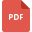# linear equations tutorialsElimination Method and Sample Problems
Mathematics, Grade 8, United States, Linear EquationsLinear and Nonlinear equations worksheet
Fundamental Math or Basic Math, Grade 8, India, Mathematical Analysis, Mathematical Logic, Linear EquationsExpressions and Equations
Expressions, Grade 4, United States, Linear EquationsEquations involving Fractions Worksheet
Linear Equations, Grade 8, United States, MathematicsSolving Two Step Equations
Mathematics, Grade 7, United States, Linear EquationsSimultaneous Linear Equations
Linear Equations, Grade 8, United States, Mathematics